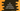# C# DivRem method explanation with examples## C# DivRem method explanation with examples

DivRem method is used to find the quotient and reminder of two numbers. It takes one output parameter and returns the reminder in that parameter. It returns the quotient.

In this post, we will learn how to use this method with examples.

## Definition of DivRem:

DivRem is defined as below:

``public static long DivRem (long dividend, long divisor, out long reminder);``

As you can see, this is a static method.

It takes dividend, divisor and reminder as the arguments and returns the quotient.

### Exception:

It throws DivideByZeroException if divisor is zero.

### Sample example:

Let’s take a look at the below example:

``````using System;

public class Program
{
public static void Main()
{
long dividend = 100;
long divisor = 10;
long reminder;
long quotient;

quotient = Math.DivRem(dividend, divisor, out reminder);

Console.WriteLine("Reminder = "+reminder+", quotient = "+quotient);

}
}``````

If you run this program, it will print:

``Reminder = 0, quotient = 10``

### Negative divisor:

We can also use a negative divisor.

``````using System;

public class Program
{
public static void Main()
{
long dividend = 133;
long divisor = -10;
long reminder;
long quotient;

quotient = Math.DivRem(dividend, divisor, out reminder);

Console.WriteLine("Reminder = "+reminder+", quotient = "+quotient);

}
}``````

It will print:

``Reminder = 3, quotient = -13``

### Exception case:

It throws exception if we try to divide by zero.

``````using System;

public class Program
{
public static void Main()
{
long dividend = 133;
long divisor = 0;
long reminder;
long quotient;

quotient = Math.DivRem(dividend, divisor, out reminder);

Console.WriteLine("Reminder = "+reminder+", quotient = "+quotient);

}
}``````

It will throw an exception:

``````Run-time exception (line 12): Attempted to divide by zero.

Stack Trace:

[System.DivideByZeroException: Attempted to divide by zero.]
at System.Math.DivRem(Int64 a, Int64 b, Int64& result)
at Program.Main() :line 12``````